# Stochastic Oscillator, the beginning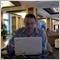Moderator
113796

I tryed to collect everything about one indicator: Stochastic Oscillator.

"Technical Analysis from A to Z":

Overview

Sto.chas.tic (sto kas'tik) adj. 2. Math. designating a process

having an infinite progression of jointly

distributed random variables.

--- Webster's New World Dictionary

The Stochastic Oscillator compares where

a security's price closed relative to its

price range over a given time period.[/CODE]

Interpretation

The Stochastic Oscillator is displayed

as two lines. The main line is called "%K."

The second line, called "%D," is a

moving average of %K. The %K line is

usually displayed as a solid line and

the %D line is usually displayed as

a dotted line.

There are several ways to interpret

a Stochastic Oscillator. Three popular methods include:

Buy when the Oscillator (either %K or %D)

falls below a specific level (e.g., 20) and

then rises above that level. Sell when

the Oscillator rises above a specific level

(e.g., 80) and then falls below that level.

Buy when the %K line rises above

the %D line and sell when the %K line

falls below the %D line.

Look for divergences. For example,

where prices are making a series of new highs

and the Stochastic Oscillator is failing to surpass

its previous highs.

[CODE]Calculation

The Stochastic Oscillator has four variables:

%K Periods.

This is the number of time periods

used in the stochastic calculation.

%K Slowing Periods.

This value controls the internal smoothing of %K.

A value of 1 is considered a fast stochastic;

a value of 3 is considered a slow stochastic.

%D Periods.

This is the number of time periods used

when calculating a moving average of %K.

The moving average is called "%D" and is

usually displayed as a dotted line on

top of %K.

%D Method.

The method (i.e., Exponential,

Simple, Time Series, Triangular, Variable,

or Weighted) that is used to calculate %D.

The formula for %K is: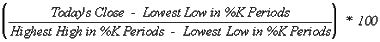For example, to calculate a 10-day %K,

first find the security's highest-high and

lowest-low over the last 10 days.

As an example, let's assume that during

the last 10 days the highest-high was 46 and

the lowest-low was 38--a range of 8 points.

If today's closing price was 41,

%K would be calculated as: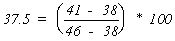The 37.5% in this example shows

that today's close was at the level of 37.5%

relative to the security's trading range over

the last 10 days. If today's close was 42,

the Stochastic Oscillator would be 50%.

This would mean that that the security

closed today at 50%, or the mid-point,

The above example used a

%K Slowing Period of 1-day (no slowing).

If you use a value greater than one,

you average the highest-high and

the lowest-low over the number of

%K Slowing Periods before performing

the division.

A moving average of %K is then

calculated using the number of time

periods specified in the %D Periods.

This moving average is called %D.

The Stochastic Oscillator always ranges between 0% and 100%. A reading of 0% shows that the security's close was the lowest price that the security has traded during the preceding x-time periods. A reading of 100% shows that the security's close was the highest price that the security has traded during the preceding x-time periods.
Files:Moderator
113796

Step stochastic indicator is on this post. This is the only correct (non-repainting, non-deformed) version of step stochastic that exist in any platform.

Divergence using Stochastic: some general information about it was collected on this thread https://www.mql5.com/en/forum/175886 and some of them are on this post.

painting the past: Stochastic is not paiting the past. But we need in some systems for this indicator to be painted the past. Read some exotic explanation/request https://www.mql5.com/en/forum/173249

how to understand the price movement using 3 Stochastic indicators:

- template attached (M5 timeframe). It was taken from asctrend M5 informational template from elite section here.

- some other informational templates about price movement understanding are here.

Multi Timeframe Stochastic:

- MTF stochastic momentum indicator is here;

- MTF Stochastic is here, and here, and here, and here, and here, and here, and here (#MTF Stochastic v. 2.0);

- Stochastic_Standard_4TF_cja updated indicator: this post.

- Many Stochastic indicators in one page collected: is here.

StochHistogram indicator is here.

- Color Stochastic indicator is here and here and here.

- StochCandles_80_20 indicator is here.

Files:Moderator
113796

Stochastic Momentum Indicator (Index):

- fixed indicator is here.

Stochastic filter indicator is here.

Stochastic Bar type MTF indicators: original thread.

On Chart Stochastic: indicator on the main window.

Stoch Signal: thread with good indicator by cja.

AllStochastics indicator: good MTF indicator developed by Igorad.

Blau's double smoothed stochastic is on this post.

Stochastic placed in chart window is on this post.Moderator
113796

EAs:

- Stoch EA is on this thread;

- Stochastic EA is here;

- Stochastic v1 EA is here;

- stoch_21 EA is here;

- Stochastic Sample EA is here;

- Stoch-Hellkas EA is here.

-StepStochCross EA. Elite section thread is here.

- Stochastic with RSI filter - StochEA: public thread.

- StoIK EA - Stoch\I-Trend: thread with few versions of the EA using i-trend indicator.

- A simple idea trading system is here;

- Stoch trading system is here and EA is here;

- ZerolagStochs system is here;

- Stochastic in BrainSystem: the thread is here and here;

- Cycles witch stochastic: elite section thread.

All John Ehlers Indicators is here (including Fisher Transform over Stochastic Oscillator, and so on).

Reverse Divergence using Stochastic indicator is here.

Stoch Signal: thread about how Stochastic's working with some good indicators is here.

Overbought/oversold condition with Stochastic: small thread with good indicators is here.1982

Thanks newdigital. Been looking at this for decads. A very complete summary for me. Again, big thank you for you.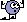Regards

David173

thanks alot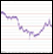96

Great topic, indeed. The stochastic indicator is presently one of my favorites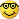It's just that it takes a while to get a real understanding of what it can do for you. For me the lights went on once I understood that you should not just use it as an overbought/-sold indicator, but also as a momentum indicator, where you take certain levels of K and/or D as indications of a trend being strong/weak, such as when being above/below 80/20.

Especially for Slow versus Fast versus Full Stochastics.

This following phrase of the first post had me wondering for a moment:

The Stochastic Oscillator always ranges between 0% and 100%.

A reading of 0% shows that the security's close was the lowest price

that the security has traded during the preceding x-time periods.

A reading of 100% shows that the security's close was the highest price

that the security has traded during the preceding x-time periods.

Since I use slow stochastics, this puzzled me as I didn't find the 100% and 0% values as the quote suggests. In fact it applies to fast stochastics, and only occasionally occurs on slow stochastics.

Attached is a chart example that should make this point immediately clear. Upper stoch is fast, lower stoch is slow.

Take care!

Files:225

I have got this picture from another topic:https://www.mql5.com/en/forum/177227, it is called TTM Stochastic and it looks very smooth and intersting.Does anybody have the formula of TTM Stoch indicator?

Shot at 2007-07-021389

ND

Thanks for the nice work. Wish there is something for all indicators....

ET17

hi mystified, i only have this one. hopely this is what are you looking for.

@ND

thx a lot ND

i think i have seen stoch with fibo line here before.did someone have it.

one more thing. what is the best setting for stoch.

i usualy use 14,3.3 for my stoch setting. does anyone use different setting.

regards

HP

Files:
ttmstoch.mq4 5 kb1259

hi

holy_phoenix:
hi mystified, i only have this one. hopely this is what are you looking for.

@ND

thx a lot ND

i think i have seen stoch with fibo line here before.did someone have it.

one more thing. what is the best setting for stoch.

i usualy use 14,3.3 for my stoch setting. does anyone use different setting.

regards

HP

For me 5,3,3 is the best ..

===================

Forex Indicators Collection